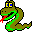#Boost C++ Libraries

...one of the most highly regarded and expertly designed C++ library projects in the world.#cuthill_mckee_ordering

Graphs: undirected color, degree time: O(m log(m)|V|) where m = max { degree(v) | v in V }
```  (1)
template <class IncidenceGraph, class OutputIterator,
class ColorMap, class DegreeMap>
OutputIterator
cuthill_mckee_ordering(const IncidenceGraph& g,
typename graph_traits<IncidenceGraph>::vertex_descriptor s,
OutputIterator inverse_permutation,
ColorMap color, DegreeMap degree)

(2)
template <class VertexListGraph, class OutputIterator>
OutputIterator
cuthill_mckee_ordering(const VertexListGraph& g, OutputIterator inverse_permutation);

template <class VertexListGraph, class OutputIterator, class VertexIndexMap>
OutputIterator
cuthill_mckee_ordering(const VertexListGraph& g, OutputIterator inverse_permutation,
VertexIndexMap index_map);

template <class VertexListGraph, class OutputIterator,
class ColorMap, class DegreeMap>
OutputIterator
cuthill_mckee_ordering(const VertexListGraph& g, OutputIterator inverse_permutation,
ColorMap color, DegreeMap degree)

(3)
template <class IncidenceGraph, class OutputIterator,
class ColorMap, class DegreeMap>
OutputIterator
cuthill_mckee_ordering(const IncidenceGraph& g,
std::deque< typename
graph_traits<IncidenceGraph>::vertex_descriptor > vertex_queue,
OutputIterator inverse_permutation,
ColorMap color, DegreeMap degree)
```
The goal of the Cuthill-Mckee (and reverse Cuthill-Mckee) ordering algorithm[14, 43, 44, 45 ] is to reduce the bandwidth of a graph by reordering the indices assigned to each vertex. The Cuthill-Mckee ordering algorithm works by a local minimization of the i-th bandwidths. The vertices are basically assigned a breadth-first search order, except that at each step, the adjacent vertices are placed in the queue in order of increasing degree.

Version 1 of the algorithm lets the user choose the ``starting vertex'', version 2 finds a good starting vertex using the pseudo-peripheral pair heuristic (among each component), while version 3 contains the starting nodes for each vertex in the deque. The choice of the ``starting vertex'' can have a significant effect on the quality of the ordering. For versions 2 and 3, find_starting_vertex will be called for each component in the graph, increasing run time significantly.

The output of the algorithm are the vertices in the new ordering. Depending on what kind of output iterator you use, you can get either the Cuthill-Mckee ordering or the reverse Cuthill-McKee ordering. For example, if you store the output into a vector using the vector's reverse iterator, then you get the reverse Cuthill-McKee ordering.

```  std::vector<vertex_descriptor> inv_perm(num_vertices(G));
cuthill_mckee_ordering(G, inv_perm.rbegin(), ...);
```

Either way, storing the output into a vector gives you the permutation from the new ordering to the old ordering.

```  inv_perm[new_index[u]] == u
```

Often times, it is the opposite permutation that you want, the permutation from the old index to the new index. This can easily be computed in the following way.

```  for (size_type i = 0; i != inv_perm.size(); ++i)
perm[old_index[inv_perm[i]]] = i;
```

### Parameters

For version 1:
• IncidenceGraph& g  (IN)
An undirected graph. The graph's type must be a model of IncidenceGraph.
Python: The parameter is named graph.
• vertex_descriptor s  (IN)
The starting vertex.
Python: Unsupported parameter.
• OutputIterator inverse_permutation  (OUT)
The new vertex ordering. The vertices are written to the output iterator in their new order.
Python: This parameter is unused in Python. The new vertex ordering is returned as a Python list.
• ColorMap color_map  (WORK)
Used internally to keep track of the progress of the algorithm (to avoid visiting the same vertex twice).
Python: Unsupported parameter.
• DegreeMap degree_map  (IN)
This must map vertices to their degree.
Python: Unsupported parameter.
For version 2:
• VertexListGraph& g  (IN)
An undirected graph. The graph's type must be a model of VertexListGraph and IncidenceGraph.
Python: The parameter is named graph.
• OutputIterator inverse_permutation  (OUT)
The new vertex ordering. The vertices are written to the output iterator in their new order.
Python: This parameter is unused in Python. The new vertex ordering is returned as a Python list.
• ColorMap color_map  (WORK)
Used internally to keep track of the progress of the algorithm (to avoid visiting the same vertex twice).
Python: Unsupported parameter.
• DegreeMap degree_map  (IN)
This must map vertices to their degree.
Python: Unsupported parameter.
For version 3:
• IncidenceGraph& g  (IN)
An undirected graph. The graph's type must be a model of IncidenceGraph.
Python: The parameter is named graph.
• std::deque< typename graph_traits<Graph>::vertex_descriptor > vertex_queue  (IN)
The deque containing the starting vertices for each component.
Python: Unsupported parameter.
• OutputIterator inverse_permutation  (OUT)
The new vertex ordering. The vertices are written to the output iterator in their new order.
Python: This parameter is unused in Python. The new vertex ordering is returned as a Python list.
• ColorMap color_map  (WORK)
Used internally to keep track of the progress of the algorithm (to avoid visiting the same vertex twice).
Python: Unsupported parameter.
• DegreeMap degree_map  (IN)
This must map vertices to their degree.
Python: Unsupported parameter.

### Example

See example/cuthill_mckee_ordering.cpp.